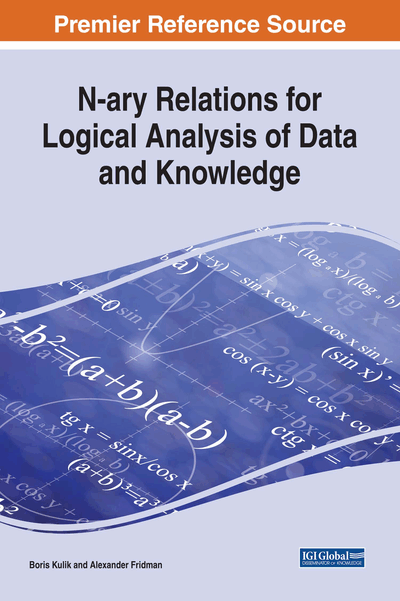# Probabilistic Modeling

Copyright: © 2018 |Pages: 30
DOI: 10.4018/978-1-5225-2782-4.ch006
OnDemand PDF Download:
Available
\$37.50
No Current Special Offers

## Abstract

Besides using logic-probabilistic analysis for probabilistic estimation itself, it is often necessary to consider uncertain or underdetermined information of a different nature expressed in the following forms: interval estimates, lists of possible options, hypothetical or possibilistic statements, etc. This chapter discusses methods of logic and logic-probabilistic analysis of uncertain data and knowledge by means of NTA. Using NTA, we can solve direct and inverse problems of probabilistic analysis of logical systems in multidimensional spaces with no limitations on classes of probability distributions.
Chapter Preview

Every natural science contains as much truth, as much mathematics it uses. – Immanuel Kant

Top

## Introduction

For a set A, the concept of a measure μ(A) is a natural extension of the following concepts (Kolmogorov & Fomin, 1999):

• 1.

The length of a segment;

• 2.

The area of a plane figure;

• 3.

The volume of a spatial figure;

• 4.

The increment f(b) – f(a) of a non-decreasing function f(t) in the half-interval [a,b);

• 5.

The integral of a nonnegative function on a linear, flat or dimensional domain.

We supplement this list with two more types of measures.

The first one is such a measure of discrete sets as the number of their elements. In the set theory, this measure is called the power (cardinality) of a set, but only powers of finite sets have all the properties of measures. Power of a set A is denoted as |A| (see Chapter 1, Relations in Mathematics and Information Systems).

The second measure (Kulik et al., 2010) is applicable to infinite sets. In the set theory, the measure of infinite sets is defined by using abstract concepts (power of a countable set, cardinality of a continuum, etc.) which do not allow to distinguish between powers of sets within one class. Thus in the class of countable infinite sets, powers of sets and their strict subsets are considered equal. For instance, the sets of natural numbers and even numbers are considered indistinguishable in the power. To prove the equivalence of an infinite set N and its strict subset Ni, a table is usually demonstrated, where each integer of N is correlated with a single number of Ni. For example, such correspondence table for non-negative integers and even numbers is as follows (Table 1).

Table 1.
Correspondence table for non-negative integers and even numbers
 N 0 1 2 3 4 5 6 7 … N2 0 2 4 6 8 10 12 14 …

## Complete Chapter List

Search this Book:
Reset